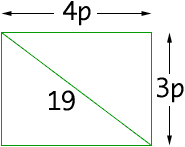Subject: ratios and area Here is my question. The aspect ratio of this rectangle is 4:3. That is A to B is 3. And B to C is 4. If I know the lenght of A to C is 19 inches, how can I find the length of A to B, and B to C. I'm not a student anymore.(way to old). But this problemĘhas been driving my crazy. I want to know the formula, so I can figure out the height and lenght of the viewing area, on all these new TVs. Sure hope you can help. Ron Delavigne Hi Ron, Since the aspect ratio is 4:3 the rectangle is 4p inches by 3p inches for some number p.By Pythagoras' theorem (4p)2 + (3p)2 = 192. That is 16 p2 + 9 p2 = 192. Hence 25 p2 = 192 and thus p = 19/5 and thus the rectangle is 3x19/5 inches by 4x19/5 inches. Cheers Penny Go to Math Central